# Object movement under the infuence of Forces on the x,y axes

Michael_0039
Homework Statement:
Mass object m is moving on plane x-y under the influence of constant force F. The projection Force on x'x is F*cos(ωt) and on y'y F*sin(ωt), ω=constant. For t=0 the Velocity=0. Find the V(t) and the route's length of the object until it stops for the first time.
Relevant Equations:
F*cos(ωt)
F*sin(ωt)
Which could be the first step to find the V(t) ?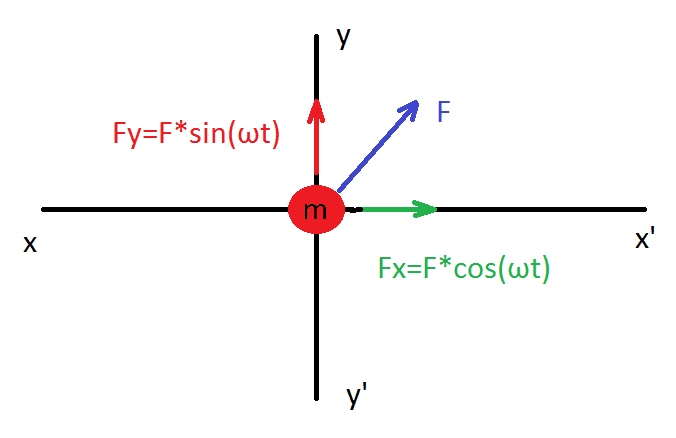Homework Helper
$$\vec F = m\vec a = m \frac {d\vec V}{dt}$$
$$(F_x, F_y) = m \frac {d} {dt} (v_x, v_y)$$

So just solve the two differential equations for the component functions ##v_x(t)## and ##v_y(t)##. Those are the components of the vector velocity ##\vec V(t)##

•Michael_0039
Homework Helper
The first step would be to find the acceleration. You could use Newton's second law.

•Michael_0039
Michael_0039
This is my try: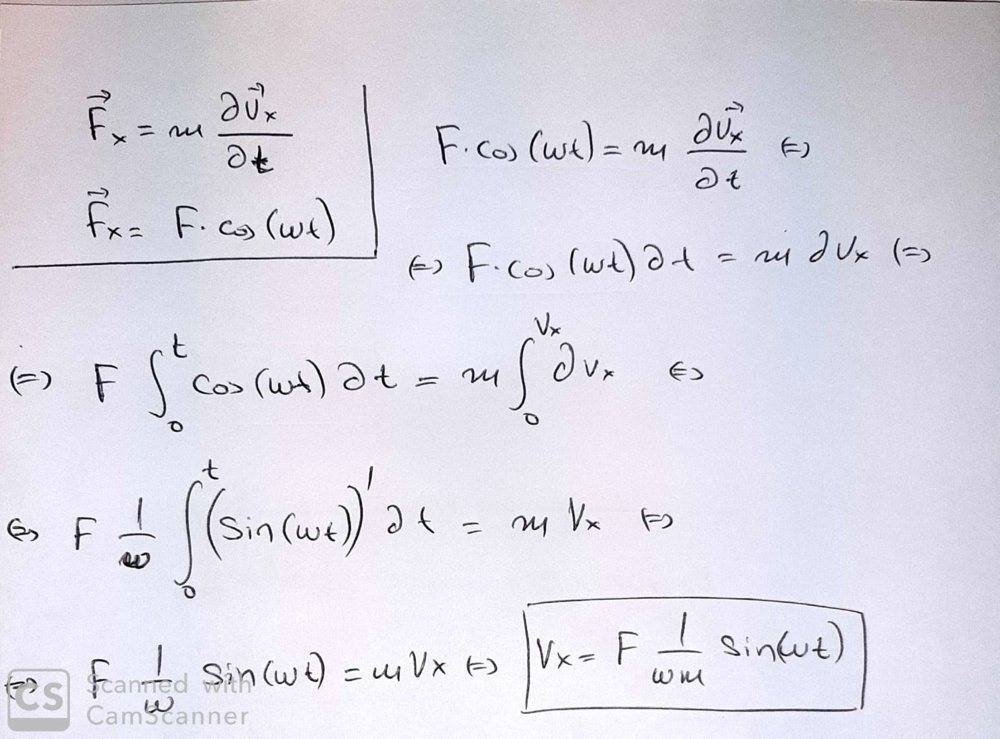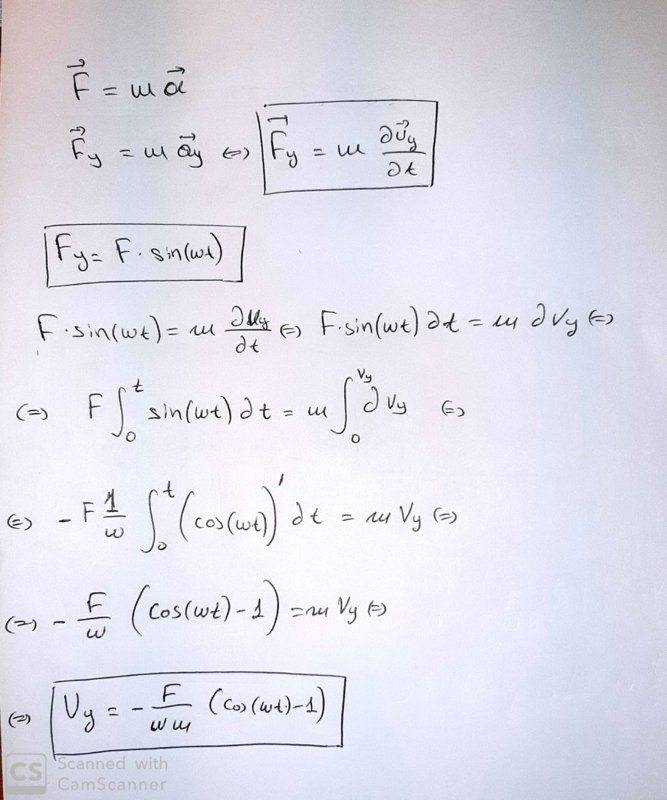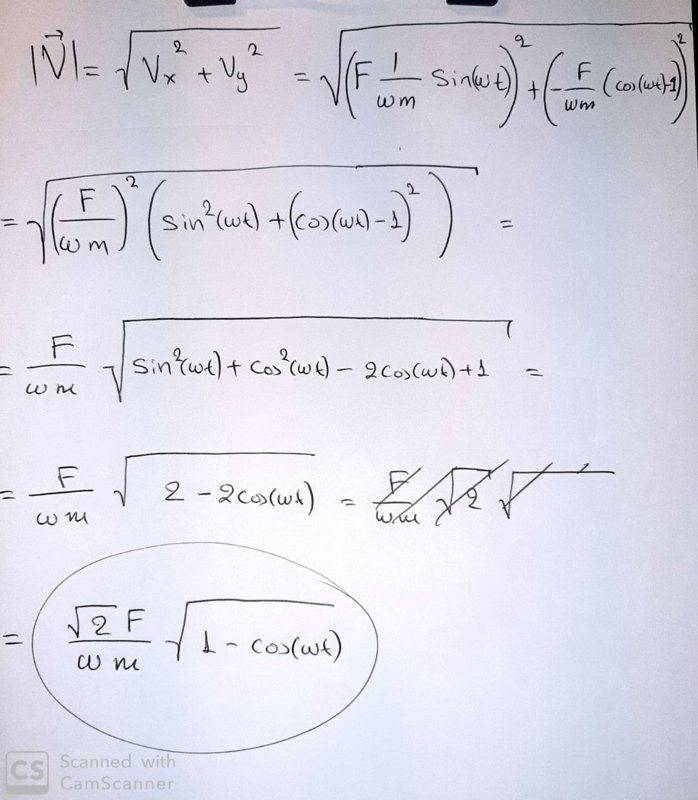•tnich
Michael_0039
Now, for the route's length, I have to integrate one more time [ ds/dt=v ]? And try to find the s. Is that process correct ?

Last edited:
archaic
Now, for the route's length, I have to integrate one more time [ ds/dt=v ]? And try to find the s. Is that process correct ?
Yeah, you have the formula ##\mathrm{ds}=|\mathbf{v}|\mathrm{dt}##.

•Michael_0039
Michael_0039
I try this, but the solution is negative, how this is explained ? And how will I work for "...until it stops for the first time "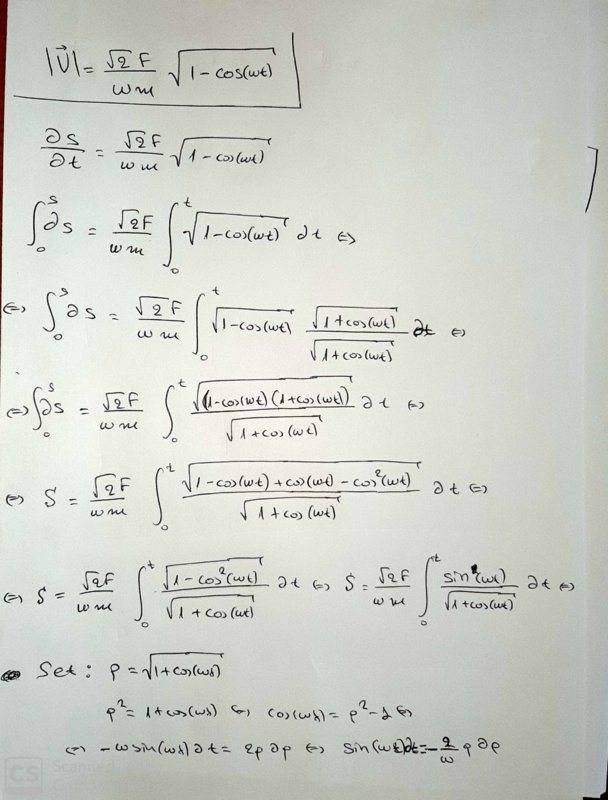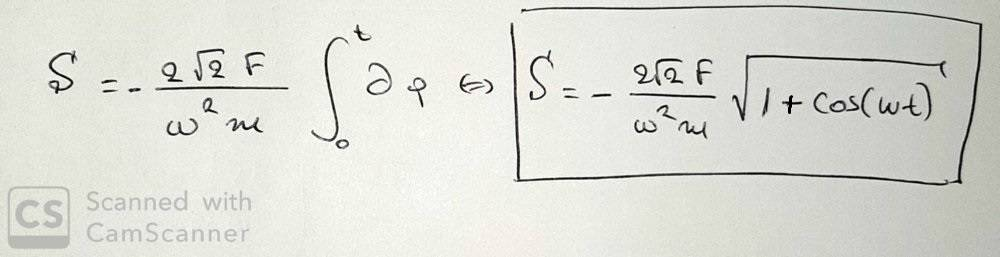Last edited:
Homework Helper
Try just integrating the velocity to get the position as a function of time. From this, the shape of the object's trajectory should be obvious, and it will allow you to easily calculated the distance traveled.

•archaic
Michael_0039
Try just integrating the velocity to get the position as a function of time. From this, the shape of the object's trajectory should be obvious, and it will allow you to easily calculated the distance traveled.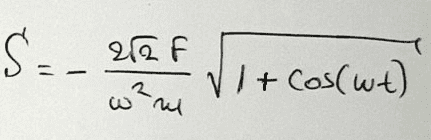but I do not understand what kind of motion describe this one?
The obvious might be a helicoidal movement, because of the Fx=F*cos(ωt) & Fy=F*sin(ωt).

archaic
View attachment 250192
but I do not understand what kind of motion describe this one?
The obvious might be a helicoidal movement, because of the Fx=F*cos(ωt) & Fy=F*sin(ωt).
you know what's weird? at ##t=0s## your object has moved a negative distance.

•jbriggs444
Homework Helper
The obvious might be a helicoidal movement, because of the Fx=F*cos(ωt) & Fy=F*sin(ωt).
You are on the right track here, but it's a 2-dimensional path. What would your helix look like if z(t) = 0?

archaic
I think your expression for ##v_y## is wrong, shouldn't it be ##v_y(t)=-\frac{F\cos{\omega t}}{m\omega}##? You have integrated between ##0## and ##t##, it should've been ##\int_{t_0}^{t'}dt##, and since velocity is zero at ##t=t_0##, you'll have ##v_y(t')=v(t')-v(t_0)=v(t')##. Same thing for ##v_x## but you've fortunate enough that ##\sin{0}=0##.

EDIT : No, I'm wrong.

Homework Helper
Gold Member
2022 Award
As @archaic points out in post #10, your expression for S does not give the right value at t=0. This is because you did not apply the bounds correctly. When you fix that you will find the constant of integration will ensure your result is positive, at least initially. But you may notice it still goes down after a while, which is also infeasible.

The next problem is that having taken the modulus of velocity to get speed, you must be careful what happens to your integral when the integrand reaches zero. Blindly integrating through that point might result in your inadvertently using negative values for speed. This is probably why the question specifies "until it comes to rest for the first time".

The whole thing becomes simpler if you get rid of the surd, which you can do by considering the half angle ##(\cos(\frac 12\omega t))##.

archaic
This is what you've done so far :
$$|\vec v|=\sqrt{v_x^2+v_y^2}=\frac{F\sqrt{2}}{\omega m}\sqrt{1-\cos{\omega t}}=\frac{2F}{\omega m}\sin{\frac{\omega}{2}t}$$
My calculation for ##S(t)## gives this, following haruspex's advice. You can see that it is zero in the beginning.
$$S(t)=\int_0^{t}|\vec v(t')|dt'=\frac{4F}{\omega^2m}(1-\cos{\frac{\omega}{2}t})$$
You probably have a stray minus sign somewhere.

•Michael_0039
Homework Helper
Gold Member
2022 Award
$$|\vec v|=\sqrt{v_x^2+v_y^2}=\frac{F\sqrt{2}}{\omega m}\sqrt{1-\cos{\omega t}}=\frac{2F}{\omega m}\sin{\frac{\omega}{2}t}$$
##\frac{2F}{\omega m}|\sin(\frac{\omega}{2}t)|##

•archaic and Michael_0039
Michael_0039
Hello all !

Now I see, using this trigonometric transformation formula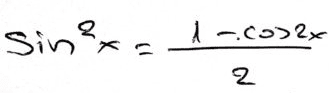, it is much better.

archaic
##\frac{2F}{\omega m}|\sin(\frac{\omega}{2}t)|##
I often miss that after rooting a square, thank you!

archaic
Hello all !

Now I see, using this trigonometric transformation formula
View attachment 250429

, it is much better.
I have something to say about your work, and I think I am right this time.
When you are looking to get the anti-derivative but without caring about the accumulation of change (to mathematically vulgarize it : ##\int_a^b df = f(a+dx)-f(a)+f(a+2dx)-f(a+dx)+...+f(b)-f(b-dx)##), you should do an indefinite integration and find the constant using given conditions.
Consider some 1D motion with a constant acceleration ##a## and an initial velocity ##v_0##.
$$\int_{t_0}^t a\,dt'=a(t-t_0)=v(t)-v(t_0)\neq v(t)=\int a\,dt=at+c\text{, }v(0)=v_0=c$$
Or ##v(t)=\int_{t_0}^t a\,dt+v(t_0)##
Someone correct me if I'm wrong!

Michael_0039
•archaic